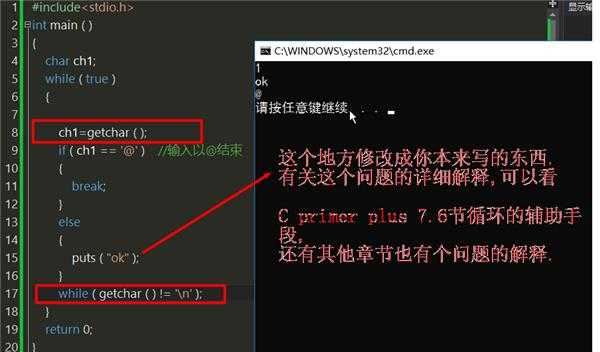﻿ 为什么这段while循环执行第二次的时候没有让本人进行键盘录入就直接跳过了 | Code Bye

# 为什么这段while循环执行第二次的时候没有让本人进行键盘录入就直接跳过了

7年前 (2017-05-03) 1282次浏览
```while(true)
{
scanf("%c",&ch1);
if(ch1=="@")    //输入以@结束
{
break;
}
else
{
Push_Seq(S1,(int)ch1);  //入栈
Push_Seq(S2,(int)ch1);   //入栈
}
}
return 0;```

2

scanf从输入缓冲区中提取字符，假如输入缓冲区为空，则等待键盘输入。

4

scanf(“%c”,&ch1);后面加上一句getchar();接收\n

3

```//请今后要用
char c;
scanf("%c",&c);
//时，都改为
char s;
char c;
scanf("%1s",s);
c=s;```

3

```//请今后要用
char c;
scanf("%c",&c);
//时，都改为
char s;
char c;
scanf("%1s",s);
c=s;```

scanf(“%1s”,s);
c=s;

scanf(“%c”,&c);

4

```#include<stdio.h>
int main ( )
{
char ch1;
while ( true )
{
ch1=getchar ( );
if ( ch1 == "@" )    //输入以@结束
{
break;
}
else
{
puts ( "ok" );
}
while ( getchar ( ) != "\n" );
}
return 0;
}```C Primer Plus中有很多关于这个问题的例子.

```while(getchar( )!="\n)
continue;```

4

Quote: 引用:

```#include<stdio.h>
int main ( )
{
char ch1;
while ( true )
{
ch1=getchar ( );
if ( ch1 == "@" )    //输入以@结束
{
break;
}
else
{
puts ( "ok" );
}
while ( getchar ( ) != "\n" );
}
return 0;
}```C Primer Plus中有很多关于这个问题的例子.

```while(getchar( )!="\n)
continue;```

``` while(isEmpty_Seq(S2)==-1)
{
Pop_Seq(S2,ch2);       //出栈
Push_Seq(S3,ch2);     //入栈
}
int isEmpty_Seq(SeqStack &S)
{
if(S.top==-1)
{
printf("栈空");
return 1;
}
else
return -1;
}```

2

CodeBye 版权所有丨如未注明 , 均为原创丨本网站采用BY-NC-SA协议进行授权 , 转载请注明为什么这段while循环执行第二次的时候没有让本人进行键盘录入就直接跳过了

[1034331897@qq.com]